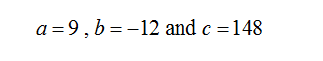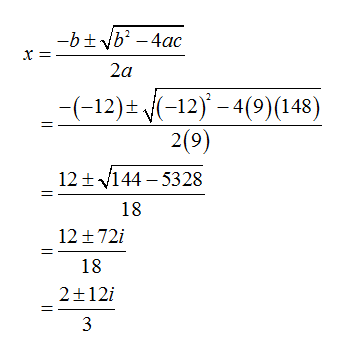# Use the Quadratic Formula to solve the equation. Use a graphing utility to verify your solution graphically. (Enter your answers as a comma-separated list.)9x2 − 12x + 148 = 0x=

Question
1 views

Use the Quadratic Formula to solve the equation. Use a graphing utility to verify your solution graphically. (Enter your answers as a comma-separated list.)

9x2 − 12x + 148 = 0

x=

check_circle

Step 1

Comparing given equation with ax2+bx+c = 0, we getNow by using quadratic formula, we have...

### Want to see the full answer?

See Solution

#### Want to see this answer and more?

Solutions are written by subject experts who are available 24/7. Questions are typically answered within 1 hour.*

See Solution
*Response times may vary by subject and question.
Tagged in